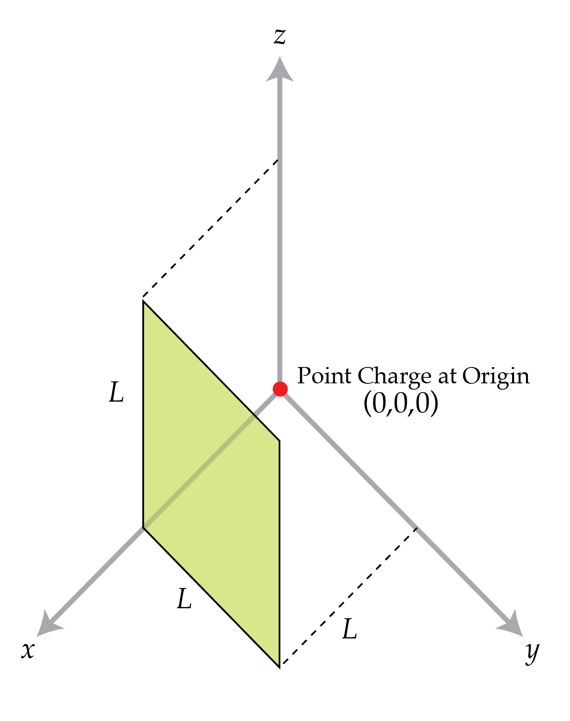# Electric flux through a square.

A flat square surface with sides of length $L$ is described by the equations $x=L, \quad 0\leq y \leq L, \quad 0\leq z \leq L.$ Find the electric flux in $\mbox{V}\cdot \mbox{m}$ through the square due to a positive point charge $q=1~\mbox{nC}$ located at the origin $(0,0,0)$.Details and assumptions

$\epsilon_{0}=8.85 \times 10^{-12}~\mbox{F/m}$

×

Problem Loading...

Note Loading...

Set Loading...